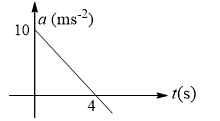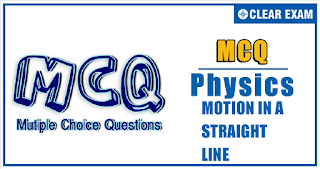## Physics Quiz-6

If an object changes its position with respect to its surroundings with time, then it is called in motion. It is a change in the position of an object over time. Motion in a straight line is nothing but linear motion. As the name suggests, it’s in a particular straight line, thus it can be said that it uses only one dimension.

Q1. An engine of a train moving with uniform acceleration passes an electric pole with velocity u and the last compartment with velocity v. The middle part of the train passes past the same pole with a velocity of
Solution
v^2-u^2=2as
Suppose velocity when middle part passes =v_m
Then v_m^2-u^2=2as×1/2=as
or v_m^2=u^2+as=u^2+(v^2-u^2)/2=(u^2+v^2)/2
⇒v_m=√((u^2+v^2)/2)

Q2.A body dropped from the top of a tower covers a distance 7x in the last second of its journey, where x is the distance covered in the first second. How much time does it take to reach the ground?
•  3 s
•  4 s
•  5 s
•  6 s
Solution
Given 7x=g/2(2n-1) and x=1/2 g(1)^2
Solving these two equations, we get n=4 s

Q3. The deceleration experienced by a moving motor boat, after its engine is cut-off is given by dv/dt=-kv^3, where k is constant. If v_0 is the magnitude of the velocity at cut-off, the magnitude of the velocity at a time t after the cut-off is
•  v_0/2
•  v
•  v_0 e^(-kt)
• v_0/√(2v_0^2 kt+1)
Solution
Here dv/dt=-kv^3
or dv/v^3 =-kdt or ∫_(v_0)^v▒dv/v^3 =∫_0^t▒〖-k dt〗
or [-1/(2v^2 )]_(v_0)^v=-kt or -1/(2v^2 )+1/(2v_0^2 )=-kt
or v^2=(v_0^2)/(1+2v_0^2 kt)  or v=v_0/√(2v_0^2 kt+1)

Q4. An elevator in which a man is standing is moving upwards with a speed of 10 ms^(-1). If the man drops a coin from a height of 2.45 m from the floor of elevator, it reaches the floor of the elevator after time (g =9.8 ms^(-2))
•  √2  s
•  1/√2  s
•  2 s
•  2 s
Solution
Let the initial relative velocity, relative acceleration and relative displacement of the coin with respect to the floor of the lift be u_r,a_r, and s_r, then
s_r=u_r t+(1/2) a_r t^2
and u_r=u_c-u_l=10-10=0
a_r=a_c-a_l=(-9.8)-0=-9.8 ms^(-2)
s_r=s_c-s_l=-2.45 m
-2.45=0(t)+(1/2)(-9.8) t^2
or t^2=1/2  or t=1/√2 s

Q5.The ratio of the average velocity of a train during a journey to the maximum velocity between two stations is
•  =1
•  >1
•  <1
•  > or <1
Solution
Since maximum velocity is more than average velocity, ratio of the average velocity to maximum velocity has to be less than one.

Q6.
 A particle moving in a straight line covers half the distance with speed of  3 m/s. The other                half of the distance is covered in two equal time intervals with speed of 4.5 m/s and 7.5 m/s              respectively. The average speed of the particle during the motion is
•  4 m/s
•  5 m/s
•  5.5 m/s
•  4.8 m/s
Solution
If t_1 and 2t_2 are the time taken by particle to cover first and second half distance respectively
t_1=(x/2)/3=x/6                                      …(i)
x_1=4.5 t_2 and x_2=7.5 t_2
So, x_1+x_2=x/2⇒4.5 t_2+7.5 t_2=x/2
t_2=x/24                                              …(ii)
Total time t=t_1+2t_2=x/6+x/12=x/4
So, average speed =4 m/sec

Q7.
Between two stations a train accelerates from rest uniformly at first, then moves with constant,
and finally retards uniformly to come to rest. If the ratio of the time taken is 1: 8: 1 and the
maximum speed attained be 60 kmh^(-1), then what is the average speed over the whole journey?
•  48 km/h
•  72 km/h
•  54 km/h
•  56 km/h
Solution
S_1=1/2 at^2=1/2 (at)t=60t/2=30t
S_2=60×8 t=480 t,S_3=S_1=30t
v_av=(S_1+S_2+S_3)/(t+8t+t)=54 km h^(-1)

Q8. A body is dropped from a height 39.2 m. After it crosses half distance, the acceleration due to           gravity ceases to act. The body will hit the ground with velocity (Take g =10 ms^(-2)):
•  19.6 ms^(-1)
•  20 ms^(-1)
•  1.96 ms^(-1)
•  196 ms^(-1)
Solution
Suppose v be the velocity of the body after falling through half the distance. Then
s=39.2/2=19.6 m,u=0 and g=9.8 ms^(-2)
v^2=u^2+2gh=0^2+2×9.8×19.6   v=19.6 ms^(-1)
When the acceleration due to gravity ceases to act, the body travels with the uniform velocity of 19.6 ms^(-1). So it hits ground with velocity 19.6 ms^(-1)

Q9.
 The acceleration-time graph of a particle moving along a straight line is as shown in Fig. At what time the particle acquires its initial velocity?•  12 s
•  5 s
•  8 s
•  16 s

• Solution
Particle will acquire the initial velocity when areas A_1 and A_2 are equal. For this, t_0=8 s

Q10. The graph below describes the motion of a ball rebounding from a horizontal surface being released from a point above the surface. Assume the ball collides each time with the floor inelasticity. The quantity represented on the y-axis in the ball’s (take upward direction as positive

•  Displacement
•  Velocity
•  Acceleration
•  Momentum
Solution
We know that for a body thrown up, its displacement is given as S=ut-1/2 gt^2. So the s-t graph is parabolic downwards.
Also the ball collides inelastically, so it will rebound to less height every time as shown in the graph
t_1,t_2,t_3 are the instants when the ball collides with ground. Here slope of the s-t graph is suddenly changing from negative to positive. It means velocity before collision is negative (downwards) and after collision is positive (upwards)#### Written by: AUTHORNAME

AUTHORDESCRIPTION## Want to know more

Please fill in the details below:

## Latest NEET Articles\$type=three\$c=3\$author=hide\$comment=hide\$rm=hide\$date=hide\$snippet=hide

Name

ltr
item
BEST NEET COACHING CENTER | BEST IIT JEE COACHING INSTITUTE | BEST NEET & IIT JEE COACHING: Motion In a Straight Line-Quiz-6
Motion In a Straight Line-Quiz-6
https://1.bp.blogspot.com/-_KLB3j4_t04/YN796ZrnaJI/AAAAAAAAQV0/6p5Bk2YWE0Qp2o09-L6Sq_jMUsUSTTwJwCLcBGAsYHQ/s320/QUIZ%2BBACK.png
https://1.bp.blogspot.com/-_KLB3j4_t04/YN796ZrnaJI/AAAAAAAAQV0/6p5Bk2YWE0Qp2o09-L6Sq_jMUsUSTTwJwCLcBGAsYHQ/s72-c/QUIZ%2BBACK.png
BEST NEET COACHING CENTER | BEST IIT JEE COACHING INSTITUTE | BEST NEET & IIT JEE COACHING
https://www.cleariitmedical.com/2021/07/motion-in-straight-line-quiz-6.html
https://www.cleariitmedical.com/
https://www.cleariitmedical.com/
https://www.cleariitmedical.com/2021/07/motion-in-straight-line-quiz-6.html
true
7783647550433378923
UTF-8

STAY CONNECTED